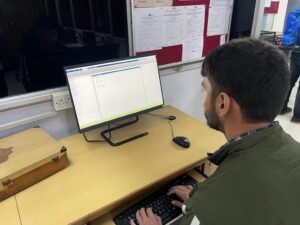Lab Room No: A308

Lab In charge: Dr. K. Sudha

Lab Attendant: Mr. Ankit Kumar

Lab Subjects: Scientific Computing Lab-ETEE 259 (III SEM)The Scientific Computing lab is a technical computing environment for high-performance numeric computation and visualization using MATLAB. The name MATLAB stands for matrix laboratory. MATLAB is a high-level programming language that has been used extensively to solve complex engineering problems. We will use MATLAB in ETEE-259 in order to illustrate the basic concepts and working of MATLAB with numerical examples. The course will utilize the MATLAB environment to provide students with a working knowledge of computer-based problem-solving methods relevant to science and engineering, including programming and numerical analysis techniques. By the end of this course, students would be able to create and apply MATLAB computer programs to analyze data and to generate tables, charts, and graphs.

Hardware Details:  10 Computer, (I-5 processor, RAM-8 GB),1 Printer

Hardware Detail: MATLAB 2017b 10 users

List of Experiment:

1. Introduction to MATLAB environment, plotting and modifying basic graphs, and evaluating basic mathematical expressions.
2. Introduction to Matrices and Vectors
3. Exploring Interactive inputs and outputs, manipulation of character strings, advanced plotting features and iterations
4. Creating and working with Functions and directories in MATLAB
5. Create an interactive function that allows the user to choose between the following. a. Display whether the user-entered number is even or odd  b.Solve and display the result of user-entered quadratic equation
6. Create an interactive function that calculates the RMS value, arithmetic mean, geometric mean and harmonic mean from the user-entered data
7. Introduction to complex numbers and advanced miscellaneous plots
8. Introduction to MATLAB Simulink, designing different circuits in Simulink and observing outputs
9. Fundamental Image Processing using MATLAB.
10. Plotting and verifying the characteristics of DC shunt motor